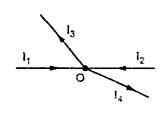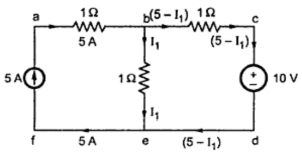### Kirchhoff's Law

In 1847, a German Physicist, Kirchhoff, formulated tow fundamental laws of electricity. These laws are of tremendous importance from network simplification point of view.
1. Kirchhoff Current law (KLC)
Consider a junction point i a complex network as shown in the Fig .1.Fig. 1
At this junction point if  I1 = 2 A ,  I2= 4 A and I3 = 1 A then to determine I4 we write, total current entering is 2 + 4 = 6 A while total current leaving is 1+ I4 A
And hence,       I= 5 A.
This analysis of currents entering and leaving is nothing but the application of Kirchhoff's Current Law. The law can be stated as,
The total current flowing towards a junction point is equal to the total current flowing away from that junction point.
Another way to state the law is,
The algebraic sum of all current meeting at a junction point is always zero
The word algebraic means considering the signs of various currents.
∑I at junction point = 0
Sign convention : Currents flowing towards a junction point are assumed to be positive while currents flowing away from a junction point assumed to be negative.
e.g. refer to Fig. 1. current I1 and I2 are positive while I3 and I4 are negative.
Applying KCL,       ∑I  at junction O = 0
I1 +I2 - I3 - I= 0  i.e.  I1 + I2 =I3 + I4
The law is very helpful in network simplification.

2. Kirchhoff Voltage Law (KVL)
"In any network, the algebraic sum of the voltage drops across the circuit elements of any closed path (or lop or mesh) is equal to the algebraic sum of the e.m.f. s in the path"
In other words, "The algebraic sum of all the branch voltages, around any closed path or closed loop is always zero."
Around a closed path   ∑V = 0
The law states that if one starts at a certain point of a closed path and goes on tracing and noting all the potential changes (either drops or rises), in any one particular direction, till the starting point is reached again, he must be at the same potential with which he started tracing a closed path.
Sum of all the potential rises must be equal to sum of all the potential drops while tracing any closed path of the circuit. The total change  in potential along a closed path is always zero.
This law is very useful in loop analysis of the network.

3. Sign Conventions to be Followed While Applying KVL
When current flows through a resistance, the voltage drop occurs across the resistance.
The polarity of this voltage drop always depends on direction of the current. The current always flows from higher potential to lower potential.
In the Fig. 2(a), current I is flowing from right to left, hence point B is at higher potential than point A, as shown.
In the Fig. 2(b), current I is flowing from left to right, hence point A is at higher potential than point B, as shown.Fig. 2

Once all such polarities are marked in the given circuit, We can apply KVL to any closed path in the circuit.
Now while tracing a closed path, if we go from - ve marked terminal to + ve marked terminal, that voltage must be taken as positive. This is called potential rise.
For example, if the branch AB is traced from A to B then the drop across it must be considered as rise and must be taken as + IR while writing the equations.
While tracing a closed path, if we go from + ve marked terminal to - ve marked terminal, that voltage must be taken as negative. This is called potential drop.
For example in the Fig. 2(a) only, if the branch is traced from B to A then it should be taken as negative, as - IR while writing the equations.
Similarly in the Fig.2(b), if branch is traced from A to B then there is a voltage drop and term must be written negative as - IR while writing the equation. If the branch is traced from B to A, it becomes a rise in voltage and term must be written positive as + IR while writing the equation.
Key point :
1) Potential rise i.e. travelling from negative to positively marked terminal, must be considered as Positive.
2) Potential drop i.e. travelling from positive to negatively marked terminal, must be considered as Negative.
3) While tracing a closed path, select any one direction clockwise or anticlockwise. This selection is totally independent of the directions of currents and voltage of various branches of that closed loop path.

4. Application of KVL to a closed path
Consider a closed path of a complex network with various branches currents assumed as shown in the Fig. 3(a).
As the loop is assumed to be a part of complex network, the branch currents are assumed to be different from each other.
Due to these currents the various voltage drops taken place across various resistances are marked as shown in the Fig. 3(b).Fig. 3

The polarity of voltage drop along the current direction  is to be marked as positive (+) to negative (-).
Let us trace this closed path in clockwise direction i.e. A-B-C-D-A.
Across R1 there is voltage drop I1 R and as getting traced from +ve to -ve, it is drop and must be taken as negative while applying KVL.
Battery E1 is getting traced from negative to positive i.e. it is a rise hence must be considered as positive.
Across R2  there is a voltage drop I2 R2 and as getting traced from +ve to -ve, it is drop and must be taken negative.
Across R there is a drop I3 R3 and as getting traced from +ve to -ve, it is drop and must be taken as negative.
Across R4 there is drop I4 R4   and as getting traced from +ve to -ve, it is drop must be taken as negative.
Battery E2 is getting traced from -ve to +ve, it is rise and must be taken as positive.
...    We can write an equation by using KVL around this closed path as,
- I1 R1 + E1 -IR -IR -IR + E = 0     ....Required KVL equation
i.e.    E+ E = I1 R +I2 R + I3 R + I4 R
If we trace the closed loop in opposite direction i.e. along A-D-B-A and follow the same sign convention, the resulting equation will be same as what we have obtained above.
Key point : So while applying KVL, direction in which loop is to be traced is not important but following the sign convention is most important.

5. Steps to Apply Kirchhoff's Law to Get Network Equations
The steps are stated based on the branch current method.
Step 1 : Draw the circuit diagram from the given information and insert all the value of source with appropriate polarities and all the resistances.
Step 2 : Mark all the branch currents with some assumed directions using KCL at various nodes and junction points. Kept the number of unknown currents minimum as far as possible to limit the mathematical calculations required to solve them later on.
Assumed directions may be wrong, in such case answer of such current will be mathematically negative which indicates the correct direction of the current. A particular current leaving a particular source has some magnitude, then same magnitude of current should enter that source after travelling through various branches of the network.
Step 3 : Mark all the polarities of voltage drops and rise as per directions of the assumed branch currents flowing through various branch resistance of the network. This is necessary for application of KVL to various closed loops.
Step 4 : Apply KVL to different closed paths in the network and obtain the corresponding equations. Each equation must contain some elements which is not considered in any previous equation.
Key point : KVL must be applied to sufficient number of loops such that each element of the network is include at least once in any of the equations.
Step 5 : Solve the simultaneous equations for the unknown currents. From these currents unknown voltages and power consumption in different resistances can be calculated.

What to do if current source exists ?
Key point : If there is current source in the network then complete the current distribution considering the current source. But while applying KVL, the loops should not be considered involving current source. The loop equations must be written to those loops which do not include any current source. This is because drop across current source is unknown.
For example consider the circuit shown in the Fig. 4. The current distribution completed in terms of current source value. Then KVL must be applied to the drop b-c-d-c-b, which does not include current source. The loop a-b-e-f-a should not be used for KVL application, as it includes current source. Its effect is already considered at the time of current distribution.Fig.  4